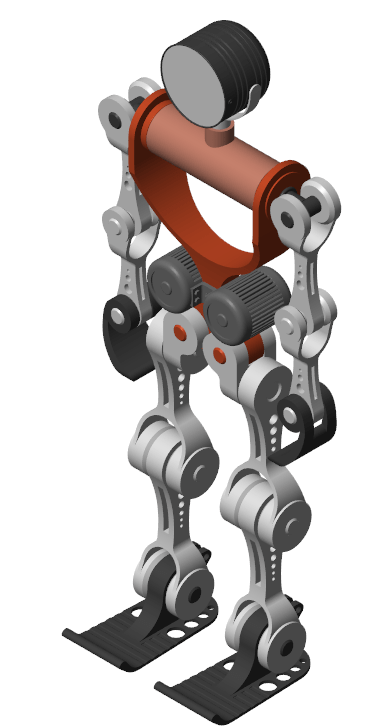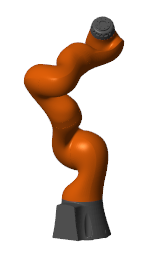# smimport

Import a CAD, URDF, or Robotics System Toolbox model

## Syntax

``````[H,dataFileName] = smimport(modelSource)``````
``___ = smimport(___,Name,Value)``

## Description

example

``````[H,dataFileName] = smimport(modelSource)``` creates a Simscape™ Multibody™ model from a CAD, URDF, or Robotics System Toolbox™ model.`modelSource` is the name of the file or object for import. Use XML files for CAD models, URDF files for URDF models, and `rigidBodyTree` (Robotics System Toolbox) objects for Robotics System Toolbox models. XML files must conform to the Simscape Multibody XML schema, and URDF files must conform to the Supported URDF Elements and Attributes. A Robotics System Toolbox license is required to create `rigidBodyTree` objects.`H` is the model handle, and `dataFileName` is the name of the supporting file that, in imported CAD models, stores the numeric values of block parameters—in a structure array populated with MATLAB variables referenced in the blocks. The data file provides a mechanism to update the imported model if the CAD model changes. Models imported from URDF files or `rigidBodyTree` objects do not rely on data files for block parameters.XML files can come from different sources. For example, the `smexportonshape` function converts CAD models into XML files. The Simscape Multibody Link plug-in is able to convert Autodesk Inventor®, PTC®, and SolidWorks® CAD models into XML files. For other CAD applications and multibody modeling tools, the Simscape Multibody XML schema makes it possible to create a custom model export app.CAD, URDF, and `rigidBodyTree` models all share the same components. These are (i) rigid bodies, also known as parts in CAD models and links in URDF models, and (ii) kinematic constraints, packaged, in some cases, as joints. Rigid bodies import as Simulink® subsystems with solid and Rigid Transform blocks. Constraints map into joint, gear, and other constraint blocks.URDF models contain `<link>` elements which in turn contain `<joint>` elements. Likewise, `rigidBodyTree` objects contain `rigidBody` objects and `rigidBodyJoint` objects. In the created Simscape Multibody model, joints become siblings to rigid bodies and features that not inside rigid body subsystems but alongside them. Joint limits and home positions persist, the later as position state targets, in the appropriate joint blocks. NoteJoint limits are imported from URDF and `rigidBodyTree` models but not from CAD models. Reproduce the joint limits of CAD models manually if you must—by enabling joint limits in the joint blocks and setting the limit positions to appropriate values. ```

example

````___ = smimport(___,Name,Value)` creates a Simscape Multibody model from a CAD, URDF, or Robotics System Toolbox model with custom name or regenerates the data file of a previously imported CAD model. Most name-value pair arguments apply only to CAD models. Use `ImportMode` to regenerate parameter data files and `PriorDataFile` to catch inadvertent changes to the model, such as the removal of a part or a change in its name. ```

## Examples

collapse all

This example shows how to create a model by importing a XML file.

To add the XML and the corresponding geometry files to the path for the current MATLAB® session, at the MATLAB® command prompt, enter:

```openExample("sm/ImportedCADModelExample"); addpath(genpath("ImportedCADModelSupport")); ```

Import a CAD Model with Default Name

To import the model and store it in memory with default name, at the MATLAB® command prompt, enter:

```smimport("sm_robot"); ```

As the model is in XML format, you can omit the file extension. The blocks in the generated model are parameterized in terms of MATLAB variables. The numerical values of these variables are defined in a data file that is named `sm_robot_DataFile.m` and stored in the same active folder as the generated model.Import a CAD Model with Custom Name

Alternatively, you can import the CAD model with a custom name, such as `robotto`. The data file is `robottos_data_file.m`.

```smimport("sm_robot","ModelName","robotto",... "DataFileName","robottos_DataFile"); ```

Regenerate the data file for the imported CAD model of the robotic arm. To avoid overwriting the original data file, name the new file `robottos_new_DataFile`.

```smimport('sm_robot','ImportMode','dataFile','DataFileName',... 'robottos_new_DataFile','PriorDataFile','robottos_DataFile'); ```

Point the imported model to the new data file and reinitialize the model workspace.

```hws = get_param(bdroot,'modelworkspace'); hws.DataSource = 'MATLAB File'; hws.FileName = 'robottos_new_DataFile'; hws.reload ```

To do the same using Model Explorer, first, select MODELING > DESIGN > Model Workspace to open the Model Explorer. Next, in the Model Hierarchy of the Model Explorer, left click Model Workspace. In the Model Workspace pane, enter `robottos_new_DataFile.m` in the File Name parameter and click Reinitialize from Source button to apply the change.

You can import a URDF model into the Simscape™ Multibody™ environment. The import process occurs in a single step based on the `smimport` function. The `smimport` function converts the URDF model directly into an equivalent Simscape Multibody model.

Example Overview

This example shows how to import an Onshape® model of a humanoid robot assembly. The model comprises various parts (”links” in URDF jargon) representing the torso, head, and limbs of the robot. The parts connect through revolute and weld joints (”continuous” and “fixed” respectively).

Import the Model

To add the URDF file to the search path for the current MATLAB® session, enter:

```openExample("sm/DocImportedURDFModelExample"); addpath(genpath("ImportedURDFSupport")); ```

Import the URDF model:

```smimport("Humanoid.urdf") ```

The function generates a Simscape Multibody model of the humanoid robot. The file extension is required to identify the import file as URDF. Update the imported model (in the Modeling tab, click Update Model.) to open a static visualization in the initial state. The figure shows the results.Build on the model, for example, by adding control systems to actuate the various joints. For a controlled example, at the MATLAB command prompt enter:

```openExample("sm/ImportedURDFExample") ```

Simulate the model to view a simple animation.

Import a `rigidBodyTree` object for an LBR iiwa serial manipulator. The model is a part of the Robotics System Toolbox installation and a URDF model named `iiwa14.urdf`.

Convert the URDF model into a `ribidBodyTree` object.

`iiwaRBT = importrobot('iiwa14.urdf');`

Note

A Robotics System Toolbox license is required to run `importrobot` (Robotics System Toolbox).

Import the `iiwaRBT` object into Simscape Multibody.

`iiwaSM = smimport(iiwaRBT);`

Update the diagram to visualize the model in its initial configuration using Mechanics Explorer. In the Modeling tab, click .## Input Arguments

collapse all

Name of import file or object, specified as a string scalar or character vector. Use XML files for CAD models, URDF files for URDF models, and `rigidBodyTree` objects for Robotics System Toolbox models. If file extension is missing, the model is assumed to be in XML format. If file path is missing, the file is assumed to be on the MATLAB® path.

Example: `'robotto.xml'`

Data Types: `char` | `string`

### Name-Value Arguments

Specify optional pairs of arguments as `Name1=Value1,...,NameN=ValueN`, where `Name` is the argument name and `Value` is the corresponding value. Name-value arguments must appear after other arguments, but the order of the pairs does not matter.

Before R2021a, use commas to separate each name and value, and enclose `Name` in quotes.

Example: smimport('sm_robot','ModelName','robotto','DataFileName','robottos_data_file');

Model topology simplification mode to use during CAD import. Set `ModelSimplification` to:

• `bringJointsToTop` to group rigidly connected parts into subsystems and promote joints to the top level in the model hierarchy.

• `groupRigidBodies` to group rigidly connected parts into subsystems but leave joints in their original places in the model hierarchy.

• `None` to import the model as is, without simplification.

Joints brought to the top level of a model are renamed using generic names based on the joint type—for example, `Revolute_Joint1`. Subsystems of rigidly connected components that have been grouped together are given generic names based on the string `RigidSubsystem`—for example, `RigidSubsystem1`. This argument applies only to CAD import.

Example: `'ModelSimplification','bringJointsToTop'`

Data Types: `char` | `string`

Option to generate a new model or update existing model data. Set `ImportMode` to `modelAndDataFile` to generate a new model and data file. Set `ImportMode` to `dataFile` to generate a new data file for a previously imported model. The function does not update the block diagram itself. If you do not specify `ImportMode`, the function runs in `modelAndDataFile` mode. This argument applies only to CAD import.

Example: `'ImportMode','dataFile'`

Data Types: `char` | `string`

Name of the Simscape Multibody model, specified as the comma-separated pair consisting of `'ModelName'` and a character vector or string scalar. The model is saved in SLX format. This argument is not valid when `ImportMode` is set to `dataFile`. If you do not specify `ModelName`, the model file is named after the multibody description file. If the multibody description file name is inconsistent with MATLAB naming rules, a slightly modified version is used instead.

Example: `'ModelName','robotto'`

Data Types: `char` | `string`

Name of the supporting parameter data file, specified as the comma-separated pair consisting of `'DataFileName'` and a character vector or string scalar. The data file is an M file with the block parameter values referenced in the imported Simscape Multibody model. If you do not specify `DataFileName`, the data file is named after the multibody description file. If the multibody description file name is inconsistent with MATLAB naming rules, a modified version is used instead. This argument applies only to CAD import.

Example: `'DataFileName','robottos_new_data'`

Data Types: `char` | `string`

Name of the last parameter data file, specified as the comma-separated pair consisting of `'PriorDataFile'` and a character vector or string scalar. The prior data file helps to identify changes requiring special attention, such as new physical units, added and deleted components, and model topology changes. This argument is valid only when `ImportMode` is set to `dataFile`. This argument applies only to CAD import.

Example: `'PriorDataFile','robottos_original_data'`

Data Types: `char` | `string`

Name of the MATLAB data structure provided in the parameter data file, specified as the comma-separated pair consisting of `'VariableName'` and a character vector or string scalar. This structure contains the numerical values of all block parameters in the Simscape Multibody model. If you specify neither `PriorDataFile` nor `VariableName`, the data structure is named `smiData`. If you specify `PriorDataFile` but not `VariableName`, the data structure name is derived from the prior data file. This argument applies only to CAD import.

Example: `'VariableName','robottosData'`

Data Types: `char` | `string`

## Output Arguments

collapse all

Simscape Multibody model, returned as a model handle. Use the model handle to get or set model parameters, for example, using the `get_param` and `set_param` functions.

Data Types: `double`

Name of the parameter data file, returned as a character vector. The file is an M file with the block parameter values referenced in the imported Simscape Multibody model. This output applies only to CAD import.

Data Types: `char`

## Version History

Introduced in R2012b